# Magnetism

#### Properties of magnetism

- The end of a magnet is called the pole.

- If a light magnet hangs on a string, one end points towards the North. This end is called the North pole.

- The other end points towards the South. This end is called the South pole.

- The same poles (North and North pole, South and South pole) repel. It is called ‘Like poles repel’.

- The different poles (North and South pole) attract. ‘Unlike poles attract’.

- Magnets attract some materials, e.g. iron, cobalt, nickel, steel… These materials that can be attracted to a magnet are called magnetic materials. Other materials, e.g. copper, plastic, paper, wood, that can’t be attracted to a magnet are called non-magnetic materials.

#### Induced magnetism

Induced magnetism is the temporary magnetisation of a magnetic material when it is placed near to or in contact with a magnet.

- If a nail is placed in contact with a permanent magnet, the nail is magnetised. And it also attracts another nail.

- The end of a nail nearer (connected) to the North pole of a permanent magnet becomes the induced South pole, and the other end becomes the induced North pole.

#### Magnetic materials (Iron and Steel)

Iron and steel are common magnetic materials. But they have different properties as shown below.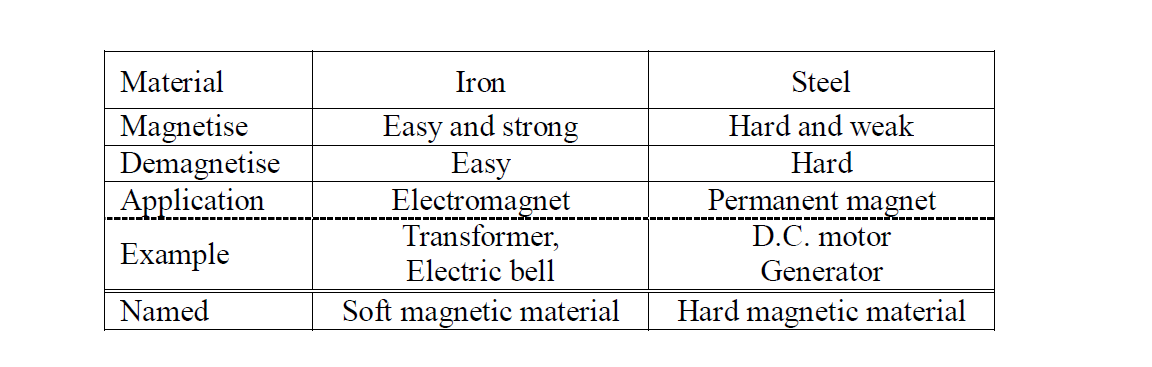If iron and steel are placed in contact with a permanent magnet, they are induced. Then, they attract some small nails. Iron attracts more nails than steel. And then, if they are removed from the permanent magnet, iron releases the nails soon but steel still attracts some nails.

#### Magnetise

##### How to make a permanent magnet
###### Stroking method

If permanent magnets are stroked along a hard magnetic material, the hard magnetic material is magnetised and it is changed into a permanent magnet.

###### Electrical method

If a hard magnetic material is palaced into a solenoid (Direct current), a hard magnetic material is magnetised and it is changed into a permanent magnet.

- This is the best method to make powerful magnets.

- The magnet which is made with a solenoid is called the electromagnet .

#### Demagnetise

###### Heating

- If a magnetised material (magnet) is heated to a higher temperature, it will lose its magnetism very quickly.

###### Hammering

If a magnetised material (magnet) is hammered many times, the magnetism becomes weaker and weaker.

###### Use alternating current and the solenoid

A magnet is placed inside a solenoid which has an A.C. supply. When the magnet is withdrawn far away from the solenoid, this process is repeated, the magnet is demagnetised.

###### Electromagnet

Iron is commonly used as the core of electromagnets because it is easy to magnetise and also demagnetise. ( It is easy to control the magnetism.)

The strength of magnetism depends on

- the current

- the number of turns per unit length of the solenoid

- the material of core

#### Magnetic field

Magnetic field is defined as the region around a magnet where magnetic effect can be detected

#### Electromagnetic effect

###### Fleming’s left-hand rule

If a current flows in a wire, in a magnetic field, a force acts on the wire.

If any electrical conductor (e.g. copper wire) move in the magnetic field and cut the magnetic flux, an e.m.f. is induced in the conductor. This is called Electromagnetic induction.

The strength of the induced e.m.f. is proportional to the rate of change of the magnetic flux.

- This law is called Faraday’s law of electromagnetic induction

#### Simple A.C. generator

- A.C. Generators generate alternate current by electromagnetic induction.

- Alternate current is the current which changes its direction a number of times per second.

- The structure of a simple A.C. generator is shown in the diagram.

- The components and functions are shown below.

- Coil produces electricity by electromaagnetic induction.

- Permanent magnets produce the magnetic field.

- Carbon brushes keep the contact with the rings continuously. Then coil is electrically connected to the outer circuit.

- Slip rings are fixed to the coil.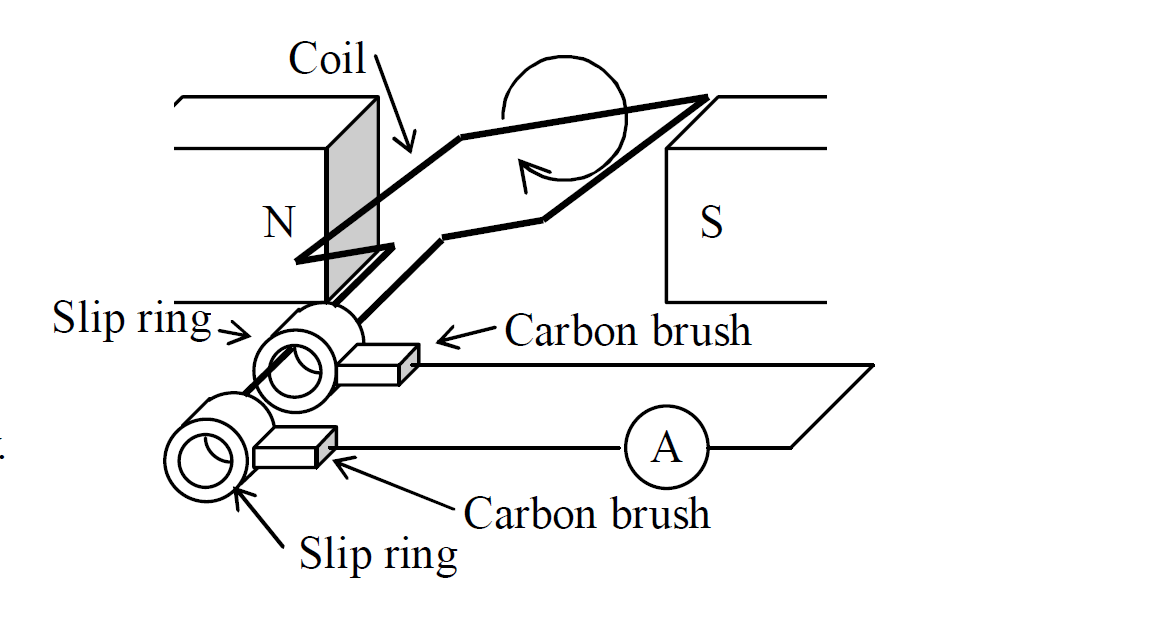#### To increase the induced current(e.m.f.)

- the coil should rotate faster.

- a stronger magnet should be used.

- the number of turns in the coil should be increased

- the coil should be wound around a soft iron core.

#### Transformer

Transformer is a device used to vary the voltage of an a.c. supply.

When the electricity is generated at the power plant, its voltage is higher than the useful voltage for our houses. Some transformers are connected between the power plant and our houses, and vary the voltage of supply from the plant to the houses.

Each country has each voltage of a.c. supply for the houses.

A transformer consists of a primary coil, a secondary coil and a soft iron core.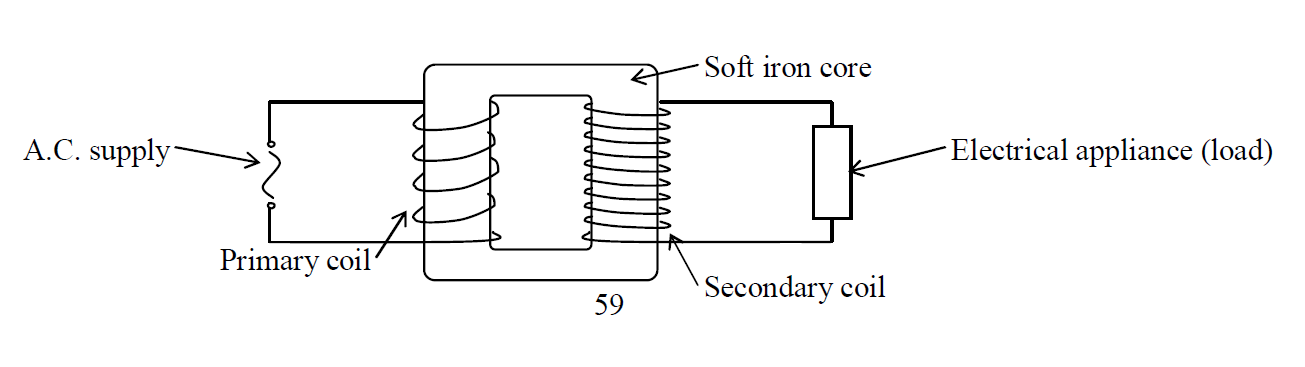The voltage of secondary coil is decided by the voltage of primary coil, the number of turns in the primary coil and the number of turns in the secondary coil. The formula is given as below.

Formula

$\frac{\mathrm{Vs}}{Vp}=\frac{\mathrm{Ns}}{Np}$

Vs: Voltage of secondary coil [V]

Vp: Voltage of primary coil [V]

Ns: Number of turns in secondary coil [Turns]

Np: Number of turns in primary coil [Turns]

#### Step-up transformer

- The voltage in the secondary coil (output voltage) is higher than the voltage in the primary coil (input voltage).

- The number of turns in the secondary coil is greater than the number of turns in the primary coil.

#### Step-down transformer

- The voltage in the secondary coil (output voltage) is lower than the voltage in the primary coil (input voltage).

- The number of turns in the secondary coil is fewer than the number of turns in the primary coil.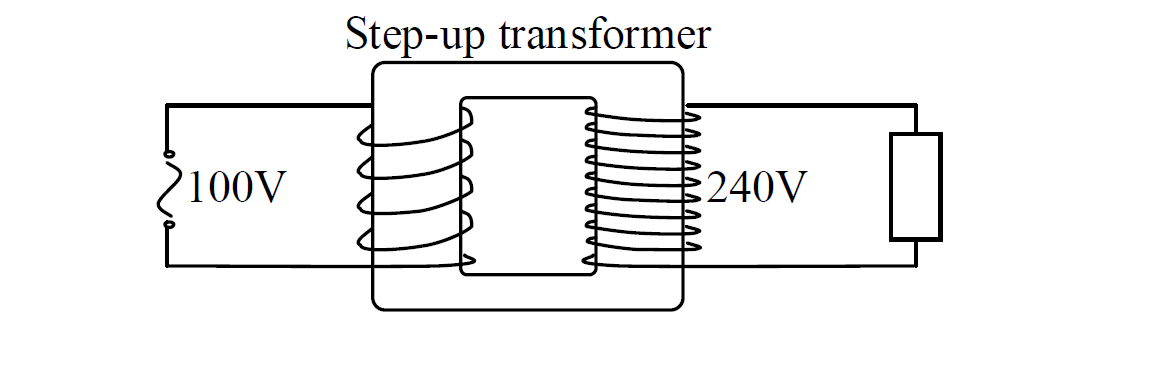#### Step-down transformer

- The voltage in the secondary coil (output voltage) is lower than the voltage in the primary coil (input voltage).

- The number of turns in the secondary coil is fewer than the number of turns in the primary coil.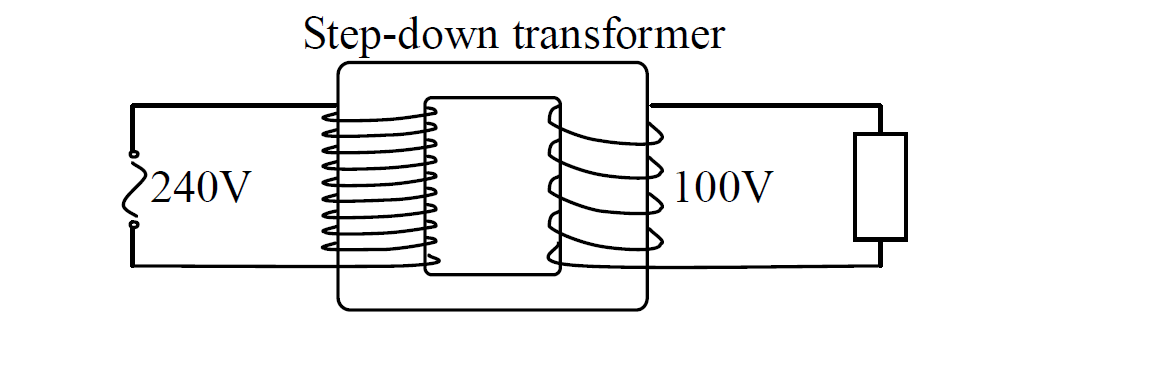If a tronsformer has the efficiency of 100% (called the ideal transformer),

Formula

$\frac{\mathrm{Vs}}{Vp}=\frac{\mathrm{Ip}}{Is}$

- Vs: The voltage of secondary coil [V]

- Vp: The voltage of primary coil [V]

- Is: The current in the secondary coil [A]

- Ip: The current in the primary coil [A]

#### Example

A step-up transformer increases the voltage of a.c. supply from 110V to 220V. The primary coil dissipates the power of 1.1kW. And the efficiency of transformer is 100%.

(a) If the turns in the primary are 400, how many turns are in the secondary?

(b) How much current flows in each coil?

Solution

(a)

$\frac{\mathrm{Vs}}{Vp}=\frac{\mathrm{Ns}}{Np}$

Given that

Vp = 110V

Vs = 220V

Np =400

Ns = ?

$\frac{\mathrm{220}}{110}=\frac{\mathrm{Ns}}{400}$

$\mathrm{110Ns}=400×220$

$\mathrm{110Ns}=88000$

$\frac{\mathrm{110Ns}}{110}=\frac{88000}{\mathrm{110}}$

$\mathrm{Ns}=800urns$

(b)

$\mathrm{Pp}=VpIp$

Given that

Vs = 220V

Pp = 1.1kW = 1100W

Vp = 110V

Ip = ?

Is = ?

$\mathrm{1100}=110×Ip$

$\mathrm{1100}=110Ip$

$\frac{\mathrm{110Ip}}{110}=\frac{\mathrm{1100}}{110}$

$\mathrm{Ip}=10A$

$\frac{\mathrm{Vs}}{Vp}=\frac{\mathrm{Ip}}{Is}$

$\frac{\mathrm{220}}{110}=\frac{\mathrm{10}}{Is}$

$\mathrm{220Is}=\mathrm{10}×1100$

$\mathrm{220Is}=11000$

$\frac{\mathrm{220Is}}{220}=\frac{11000}{\mathrm{220}}$

Answer: $\mathrm{Is}=5A$